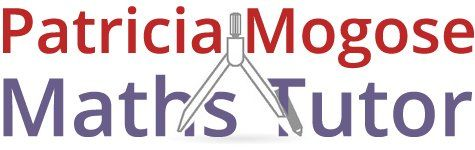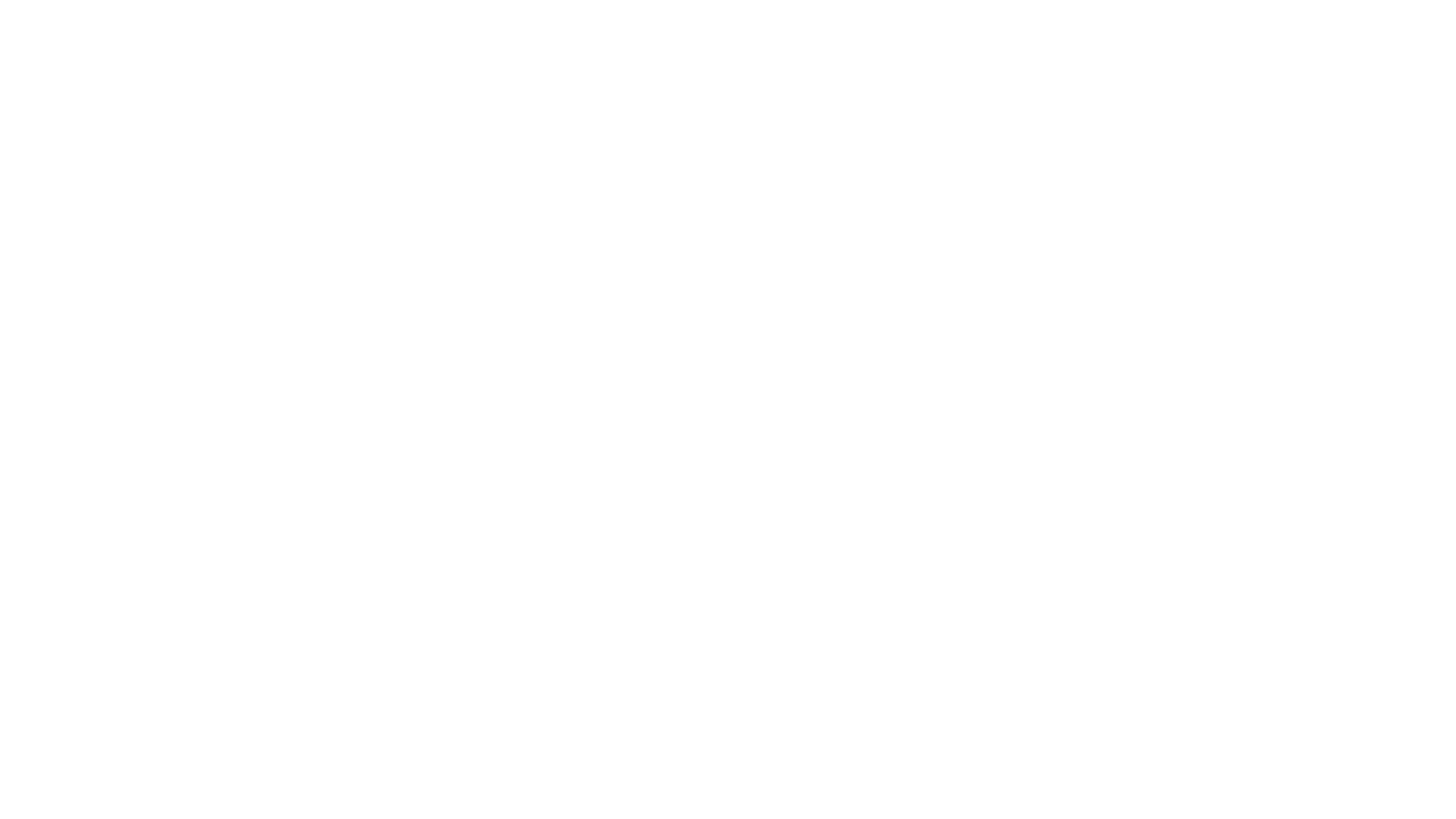For maths tuition with Patricia, call:
01733 265 440
patricia.mogose@sky.com

# Preparation for GCSE and A-level examsin Peterborough

Whether you are preparing for GCSE or A-level exams, you can rely on t Patricia Mogose Maths Tutor in Peterborough. I offer personalised maths tuitions at affordable rates. You can also contact me for primary and secondary school maths tuition.

#### BSc Honours and PGCE qualified### The Foundation level covers:

Foundation tier students sit the GCSE exam at the end of Year 11 and exam grades fall between 1 – 5. They will take 3 exams, one of which is a non-calculator paper, and the the other 2 are calculator papers. The exam will focus on material learnt in previous years, as well as the new work they will cover in the last 2 years, as follows:

• Standard Index form: mental and calculator
• Working with fractions in ratio problems
• Find exact solutions in terms of pi
• Use inequality notation to express error intervals
• Understand limits of accuracy in calculations
• Substitute numerical values into expressions and forrmulae
• Recognise expressions, equations, formulae, terms and identities
• Work with surds
• Show algebraic expressions are equivalent
• Factorising simple expressions
• Expanding double brackets
• Solving equations with negative solutions
• Find approximate solutions and turning points of a quadratic function graphically
• Recognise cubic and reciprocal functions
• Work with graphs involving speed, distance, time and acceleration
• Extend sequences to recognise squares, cubes, Fibonacci-type and simple geometric sequences
• Use compound units of speed, density, pressure and rates of pay
• Percentage increase/decrease, simple interest and percentages more than 100%
• Growth, decay and compound interest
• Work with direct and inverse proportion
• Constructions and loci using compass and ruler
• Transformations: rotation by angle and direction, reflection in specified line, translation by vector, enlargement by scale factor, maybe fractional and the centre
• Circle: sector, segment, tangent and chord, arc length and sector area
• Plan and elevation of 3D shapes
• vectors: addition, subtraction, simple multiplication and graphical illustration
• Probability: relative frequency, combined events and tree diagrams
• Venn diagrams
• Statistics: samples and populations
• Pie charts, line graphs, scatter graphs, frequency diagrams, stem and leaf diagrams
• Interpret and calculate various statistical measures### Higher level topics:

Higher tier students sit the GCSE exam at the end of Year 11 and exam grades fall between 4 – 9. They will take 3 exams, one of which is a non-calculator paper, and the the other 2 are calculator papers. The exam will focus on material learnt in previous years, as well as the new work they will cover in the last 2 years. This will include all material described in the Foundation tier, and additional material as follows:

• Estimation of powers and roots for any positive number
• Work with fractional indices
• Further surd work involving rationalising a denominator
• Find the exact conversion of a recurring decimal as a fraction
• Determine upper and lower bounds of a calculation
• Simplify algebraic expressions involving surds and fractions
• Factorising more complex quadratic expressions
• Prove mathematically that 2 expressions are equivalent
• Inverse functions
• Identify and find equations for perpendicular and parallel lines
• Determine the turning point of a quadratic by completing the square
• Exponential and trigonometric graphs
• Transformation of graphs
• Areas under graphs
• Equation of a circle and of a tangent to a circle
• Solve quadratic equations by formula and square completion
• Solve simultaneous equations, one linear, one quadratic
• Approximate the solution to an equation by iteration